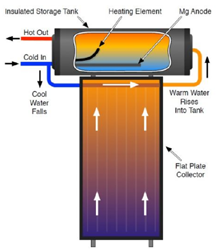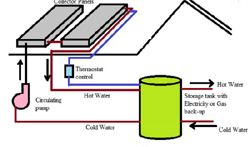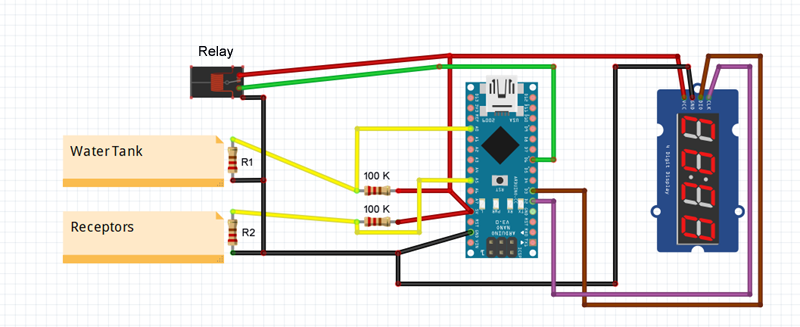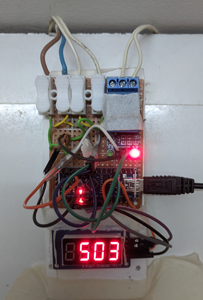# Share My CreationSolar Heating - circulating pump control

#### derez

##### Expert
Something that worked for more than 40 years broke and I had to find a replacement. I want to show what I did.
Background:
Solar heating systems (for houses) can either be autonomous, where the water circulation is done by itself - hot water go up because it is lighter than the cold. Here the tank is above the receptors.Where the tank is below the receptors, you need to circulate the water with a pump. You don't want to circulate it all the time, only when the water in the receptor is warmer than what is in the tank.
For this there is a controller (that broke down in my system).The controller compares the resistance of two thermistors which are inside the receptors and the tank and turns a relay on or off.

Implementation:
See post #5 for an improvement !

I checked the resistance of the two thermistors and it was about 70K ohm, so I connected each to 100K ohm resistor, and measured the middle point.
The measured analog value when connected to an "A" pin is 1023 * R1/(100k+R1) and the same for R2.
I used Arduino Nano.
I added a 4 digits & segment to show the value all the time for calibration.
In the code I measure both values every 5 seconds and calculate the mean of the difference every 30 seconds , showing this result in the display.
I could not get negative numbers to show correctly so I added 500 to every result.This is how it actually looks, the relay pins are covered for protection - there is 220v there, removed just for the photo. I'll probably put it all in a nice box when I'll get a
round tuit...For calibration I checked the result when the time was right to turn on the pump and when it should be turned off and set these limits in the code:
B4X:
``````Sub Process_Globals
'These global variables will be declared once when the application starts.
'Public variables can be accessed from all modules.
Public Serial1 As Serial
Private roof, boiler, relay As Pin
Private tmr As Timer
Private tm As TM1637Display
Private count As UInt = 0
Dim dif As Int

End Sub

Private Sub AppStart
Serial1.Initialize(115200)
Log("AppStart")
roof.Initialize(roof.A0, roof.MODE_INPUT)
boiler.Initialize(boiler.A5, boiler.MODE_INPUT)
tmr.Initialize("tmr_Tick", 5000)
tmr.enabled = True
tm.Initialize(2,3)

relay.Initialize(6,relay.MODE_OUTPUT)  ' needs logic converter to work, need 5v
relay.DigitalWrite(True)

End Sub

Sub tmr_tick
count = (count + 1) Mod 6

Dim b,r As Int

dif = dif + r - b + 500  ' +500 to eliminate negative numbers
Log(r , "  ",b, "  ",dif )

If count = 0 Then
dif = dif/6
Log(dif)
tm.ShowNumberDec(dif)
If dif < 300 Then
relay.DigitalWrite(False)
Log("switch on")
else If dif > 350 Then
relay.DigitalWrite(True)
Log("switch off")
Else
Log("no change")
End If
dif = 0
End If
End Sub``````

Last edited:

#### Peter Simpson

##### Expert
Ooh nice, very nice indeed @derez.

#### Acuario

##### Member
Nice simple project, I have two controllers operating using esp8266 so wifi connected but written in c++ not b4r.

Depending on where you live climate wise, it might be worth adding a frost protect subroutine. I live in a zone where it rarely drops below zero but it just takes one very cold night to freeze and split the pipes in the solar collector.

In my controller I turn on the circulating pump for a short while (a minute) if I detect the temperature is around 1 degree.